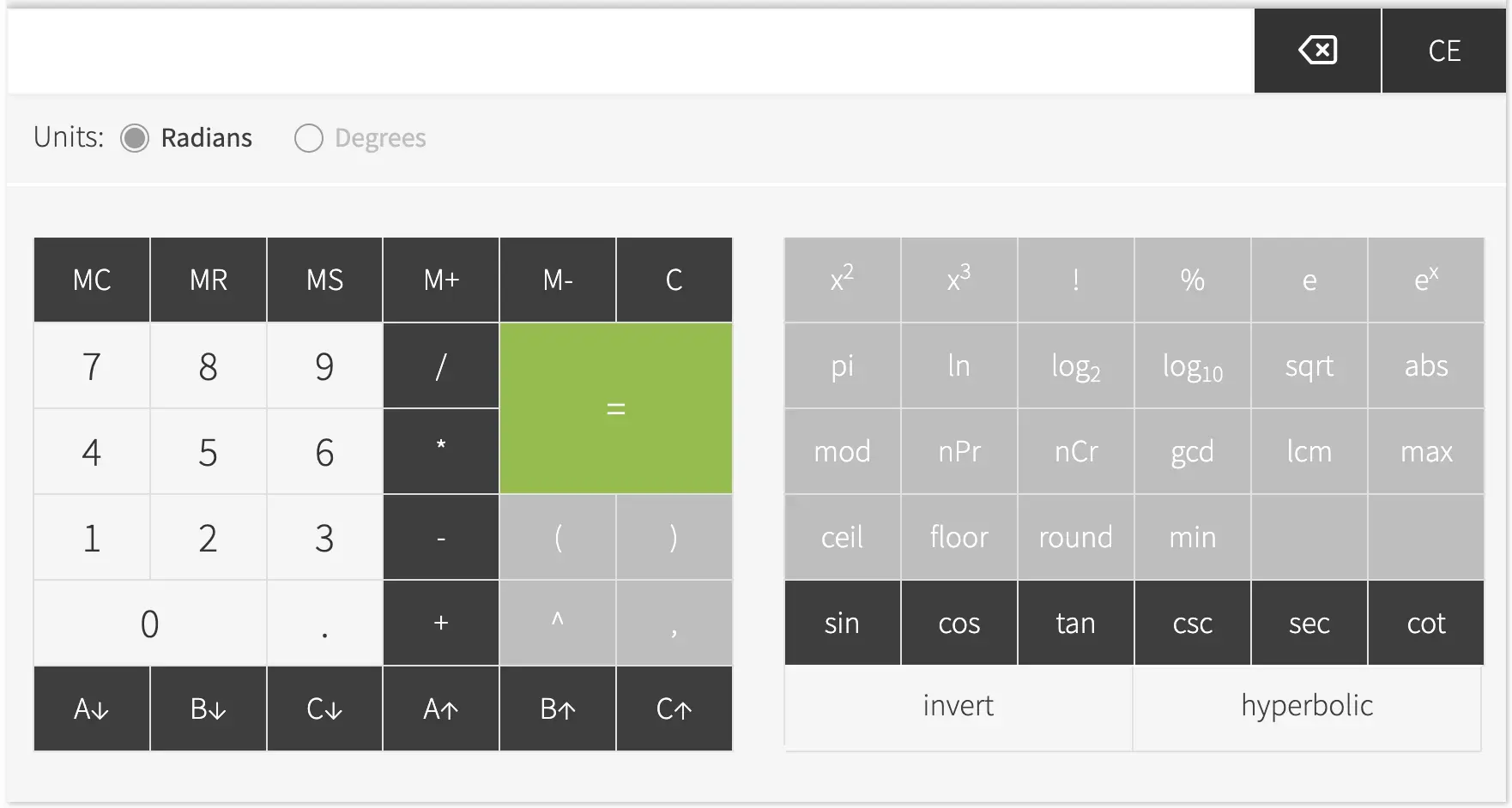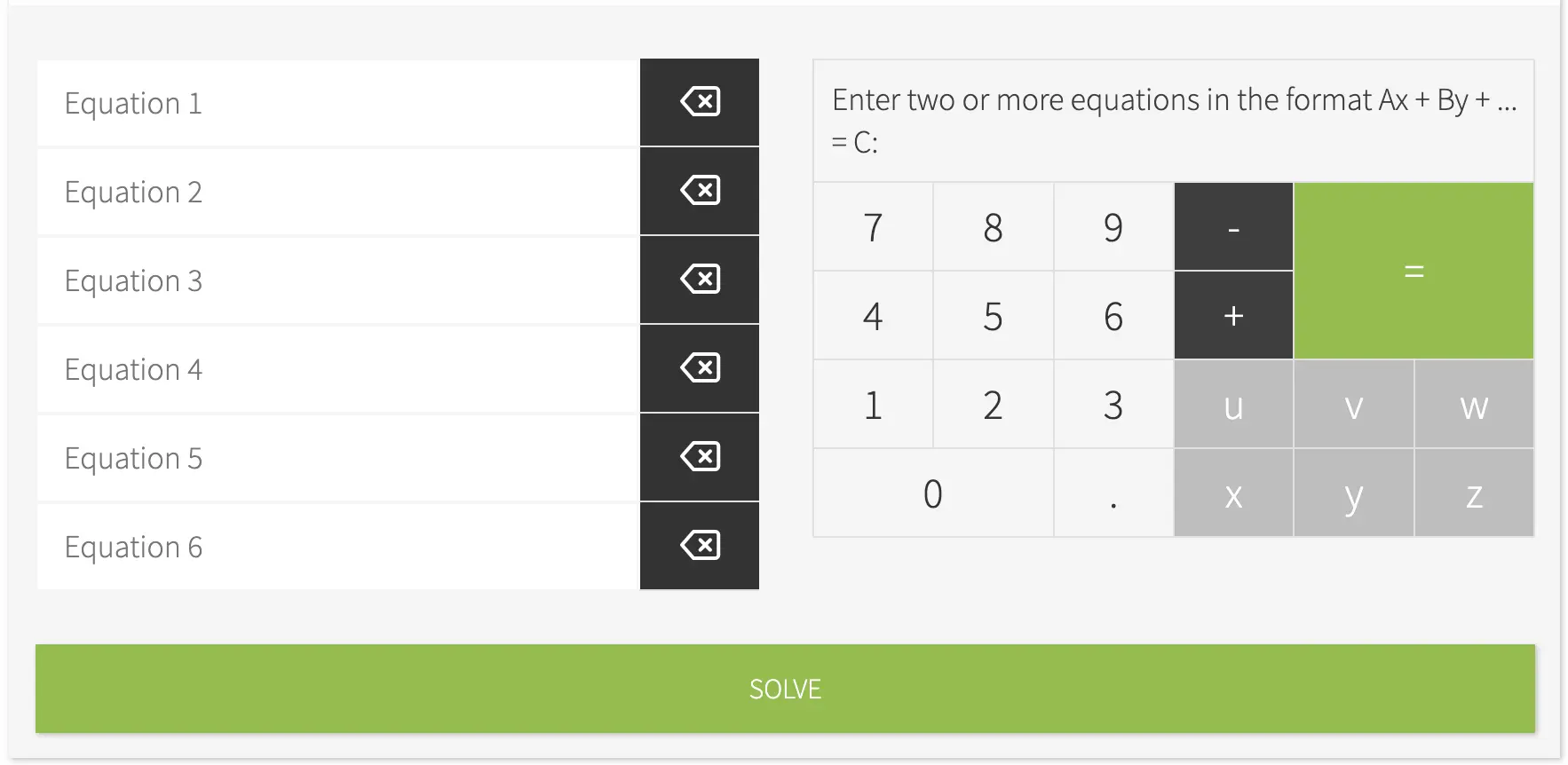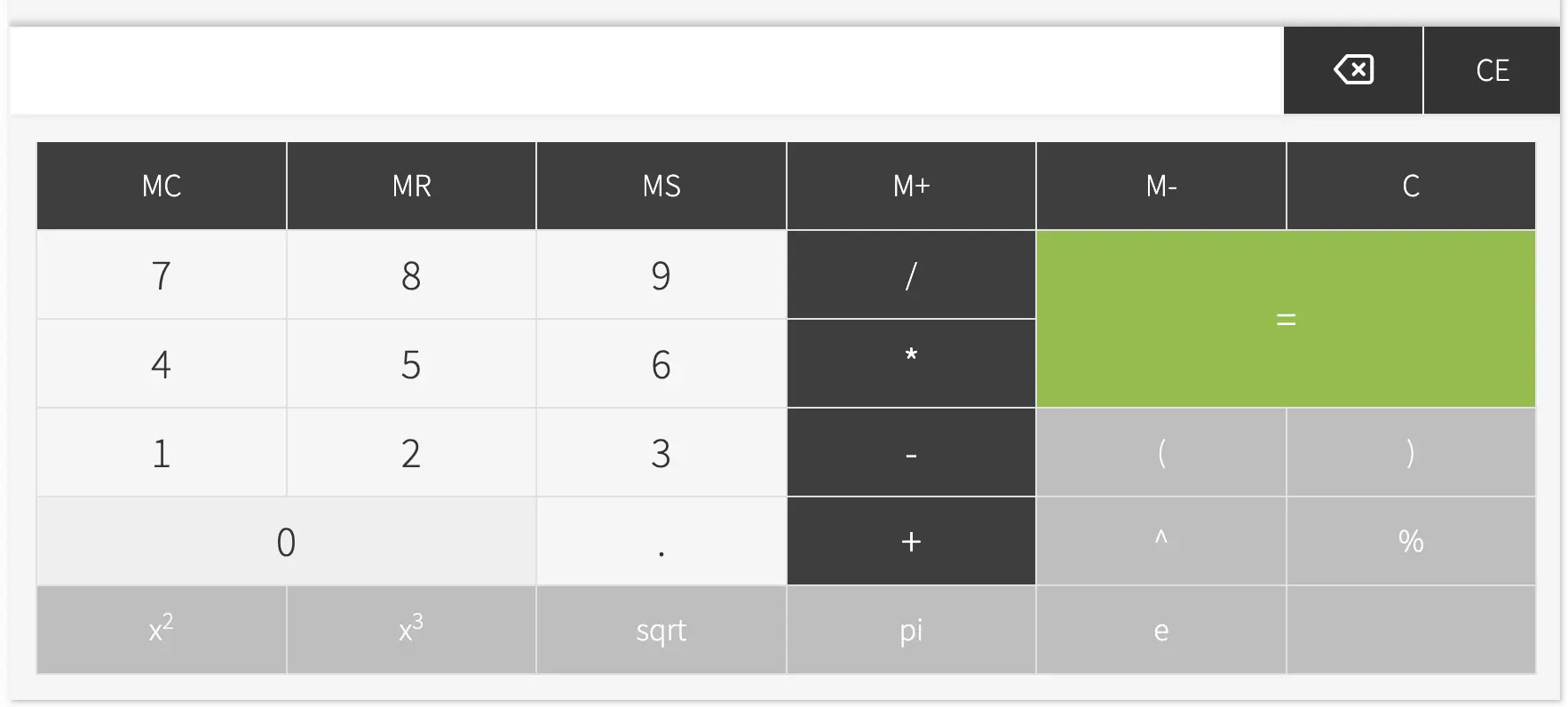# Scientific Calculator and Equation Solver

• Our scientific calculator is the most sophisticated and comprehensive scientific calculator online.
• It has all the basic functions and buttons you'd expect including sin, cos, tan, sin-1, cosh, log and much more.
• Also has some more advanced features including a button to calculate the least common multiple, permutations and combinations.
• A linear equations solver that lets you input up to 6 equations with either two or three variables and the solver will calculate the solutions.
• Memory button for storing calculations for future use.# Best Scientific Calculator Online

#### What is a scientific calculator?

A scientific calculator is a type of electronic calculator designed to perform advanced mathematical operations. This calculator can also perform basic arithmetic functions just like simple calculators. If you are in a field related to engineering, mathematics, and science, you will likely need a scientific calculator to solve complicated math problems. The good news is that you can now access a myriad of its features using our free online scientific calculator.

Our online scientific calculator is comprehensive enough to help you whenever and wherever you need it. You can use this calculator to solve difficult problems in geometry, trigonometry, and logarithms. The calculator can also handle very small or very large numbers, making it best for astronomy, physics, and chemistry use.

Lastly, our calculator has a memory button for storing calculations for future use. This is especially helpful if you are working on something that requires more than one session. The memory option makes it easy to return if you need to pause or if you want to try another equation or problem.

1. The scientific calculator which has all the basic functions and buttons including sin, cos, tan, sin-1, cosh, log, and much more. Advanced features are also available including a button to calculate the least common multiple, permutations, and combinations.Figure 1. Screenshot of the Scientific Calculator

2. The equation solver which allows you to solve equations by inputting up to 6 equations with either two or three variables and the solver will calculate the solutions.Figure 2. Screenshot of the Equation Solver

3. A simple calculator that contains basic mathematical functions.Figure 3. Screenshot of the Simple Calculator